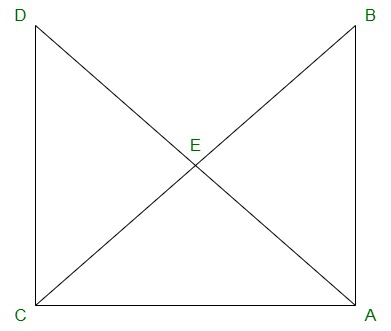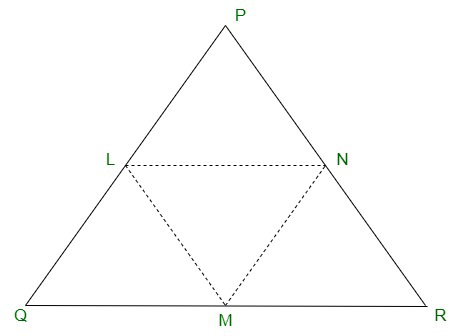GeeksforGeeks App
Open AppBrowser
Continue

## Related Articles

• RD Sharma Class 9 Solutions

# Class 9 RD Sharma Solutions – Chapter 10 Congruent Triangles- Exercise 10.4

### Question 1: In figure, It is given that AB = CD and AD = BC. Prove that ΔADC ≅ ΔCBA.Solution:

Given:
AB = CD and AD = BC.

To prove:

AB = CD {Given}
And AC = AC {Common side}
So,
By SSS congruence criterion, we have

Hence, proved.

### Question 2: In a ΔPQR, if PQ = QR and L, M and N are the mid-points of the sides PQ, QR and RP respectively. Prove that LN = MN.

Solution:Given:

In Δ PQR, PQ = QR and L, M and N are the mid-points of the sides PQ, QR and RP respectively

To prove:
LN = MN

Join L and M, M and N, N and L
We have PL = LQ, QM = MR and RN = NP
[Since, L, M and N are mid-points of PQ, QR and RP respectively]
And also PQ = QR
PL = LQ = QM = MR = PN = LR —->(equation 1)

MN || PQ and MN =  PQ    { Using mid-point theorem}
2
MN = PL = LQ —->(equation 2)

Similarly, we have
LN || QR and LN =  QR
2
LN = QM = MR —->(equation 3)

From (equation 1), (equation 2) and (equation 3),
We have
PL = LQ = QM = MR = MN = LN
This implies, LN = MN

Hence, Proved.

My Personal Notes arrow_drop_up
Related Tutorials